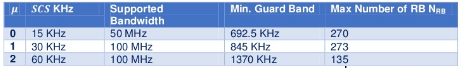# Spectral Efficiency : 5G-NR and 4G-LTE

What do you think of when you hear word “spectral efficiency?” The answer will come, it has to do with the amount of information that fits in a given channel bandwidth or one just say how efficiently can that piece of spectrum be used to transmit information.

Before we digging into the details of spectral efficiency, it’s important to understand that there is a hard limit to how much data can be transmitted in a given bandwidth and  this limit is well-known as Shannon-Hartley Theorem and commonly referred to as the Shannon limit.

Spectral efficiency usually is expressed as “bits per second per hertz,” or bits/s/Hz. In other words, it can be defined as the net data rate in bits per second (bps) divided by the bandwidth in hertz. Net data rate and symbol rate are related to the raw data rate which includes the usable payload and all overhead.

• net data rate = raw data rate – overhead
• Spectral efficiency = net data rate in bps / Channel Bandwidth in Hz

For example, a system uses channel bandwidth as 2 MHz and it can support a raw data rate of say 15 Mbps, assuming 2 Mbps as overhead then net date rate will be as 13 Mpbs, then its spectrum efficient can be calculated as follows:

• Spectral efficiency= 13 x 10^6 / 2 x 10^6 = 6.5 bits/second/Hz

Calculating Spectral Efficiency for LTE:

A LTE system can support a maximum channel bandwidth as 20 MHz (Not including Carrier Aggregation). Its symbol rate can be calculated as

• Symbols/Second = 1200 x 14 x 1000 = 16,800,000 Symbols/Second

Considering 64-QAM as highest modulation for downlink each symbol can carries 6 bits provide raw data rate  as follows:

• raw data rate = 16,800,000 x 6 = 100.8 Mbps (No MIMO considered)

Lets consider 4×4 MIMO, theoretically it makes raw data rates  four times i.e. 400 Mbps. assuming 25 % as overhead the net data rate will be as 300 Mbps. Similarly data rate can be calcuated for uplink . In LTE uplink there is not MIMO, so Max raw data can be 100 Mbps with 64-QAM support in Uplink and after deducted 25% overhead net data rate for uplink will be 75 Mbps. Uplink net date with 16-QAM will be 51 Mbps.

• Downlink Spectral Efficiency = 300 x 10^6 bps  / 20 x 10^6 Hz = 15 bits/second/Hz
• Uplink Spectral Efficiency (64-QAM UL) = 75  x 10^6 bps  / 20 x 10^6 Hz = 3.75 bits /second / Hz
• Uplink Spectral Efficiency (16-QAM UL) = 51  x 10^6 bps  / 20 x 10^6 Hz = 2.55 bits /second / Hz

Calculating Spectral Efficiency for 5G New Radio:

5G New Radio is capable of providing downlink throughput 2.31 Gbps and uplink throughput of  2.47 Gbps with certain configuration shown below with 100 MHz channel bandwidth. (Single carrier component)• Downlink Spectral Efficiency = 2.31 x 10^9 bps  / 100 x 10^6 Hz = 23 bits/second/Hz
• Uplink Spectral Efficiency = 2.47  x 10^9 bps  / 100 x 10^6 Hz = 24 bits /second / Hz

Note: The values shown here are just theoretical value considering some assumptions.

Related Post: